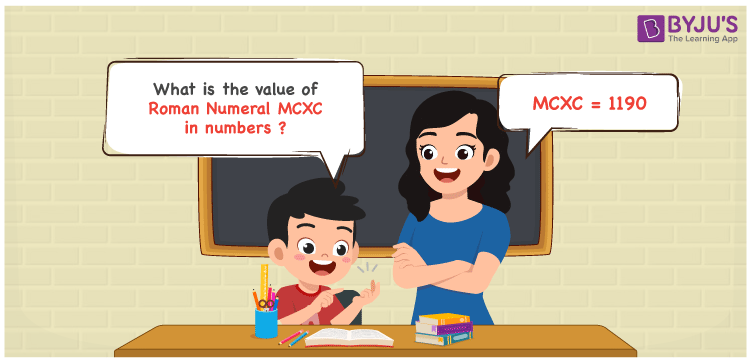Checkout JEE MAINS 2022 Question Paper Analysis : Checkout JEE MAINS 2022 Question Paper Analysis :

# MCXC Roman Numerals

MCXC Roman Numerals is 1190. Roman numerals MCXC should be expanded and with the help of the symbols chart, the respective number can be obtained using the addition operation. Students will grasp the Roman numerals concept by reading this conversion process on a regular basis. Therefore, the Roman numerals MCXC is written as 1190 in numbers.

 Number Roman Numeral 1190 MCXC## How to Write MCXC Roman Numerals in Numbers?

Learn the process of indicating roman numerals MCXC in numbers using the expansion available below.

MCXC = M + C + (C – X)

MCXC = 1000 + 100 + (100 – 10)

MCXC = 1190

## Video Lesson on Roman Numerals## Frequently Asked Questions on MCXC Roman Numerals

### Why is 1190 shown as MCXC in Roman Numerals?

We know that

MCXC = M + C + (C – X)

MCXC = 1000 + 100 + (100 – 10)

MCXC = 1190

### Find the value of 1200 – 10.

We know that

1200 – 10 = 1190

Hence, the value of 1200 – 10 is 1190 which is written as MCXC.

### What is the remainder when MCXC is divided by III?

We know that

MCXC = 1190

III = 3

The remainder when MCXC is divided by III is II.